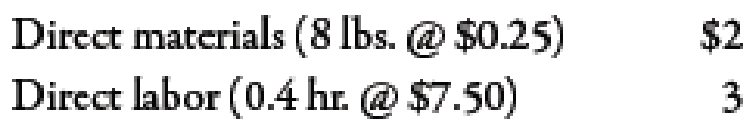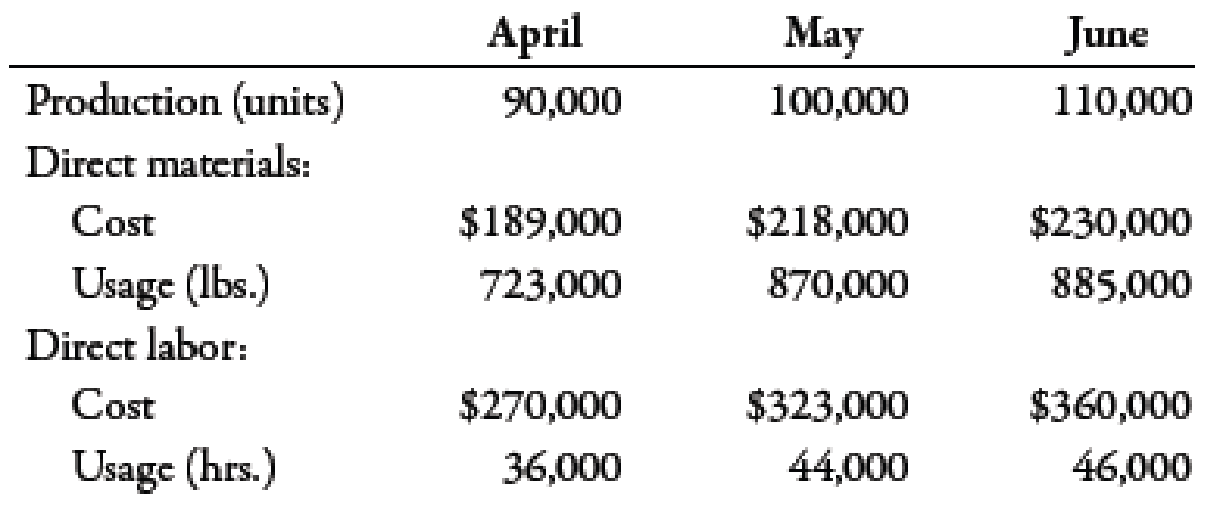Chapter 10, Problem 68P### Managerial Accounting: The Corners...

7th Edition
Maryanne M. Mowen + 2 others
ISBN: 9781337115773

#### Solutions

Chapter
Section### Managerial Accounting: The Corners...

7th Edition
Maryanne M. Mowen + 2 others
ISBN: 9781337115773
Textbook Problem
151 views

# The management of Golding Company has determined that the cost to investigate a variance produced by its standard cost system ranges from $2,000 to$3,000. If a problem is discovered, the average benefit from taking corrective action usually outweighs the cost of investigation. Past experience from the investigation of variances has revealed that corrective action is rarely needed for deviations within 8% of the standard cost. Golding produces a single product, which has the following standards for materials and labor:Actual production for the past 3 months follows, with the associated actual usage and costs for materials and labor. There were no beginning or ending raw materials inventories.Required: 1. What upper and lower control limits would you use for materials variances? For labor variances? 2. Compute the materials and labor variances for April, May, and June. Identify those that would require investigation by comparing each variance to the amount of the limit computed in Requirement 1. Compute the actual percentage deviation from standard. Round all unit costs to four decimal places. Round variances to the nearest dollar. Round variance rates to three decimal places so that percentages will show to one decimal place. 3. CONCEPTUAL CONNECTION Let the horizontal axis be time and the vertical axis be variances measured as a percentage deviation from standard. Draw horizontal lines that identify upper and lower control limits. Plot the labor and material variances for April, May, and June. Prepare a separate graph for each type of variance. Explain how you would use these graphs (called control charts) to assist your analysis of variances.

1.

To determine

Compute the upper and lower limit of materials and labor.

Explanation

Variance:

The amount obtained when actual cost is deducted from budgeted cost is known as variance. Variance is calculated to find whether the cost is over applied or under applied.

Use the following formula to calculate the value of upper limit of material for April:

Upper limit=(Direct Material Quantity×Price Standard1)+ Price Standard

Substitute 0.08 for direct material quantity and $180,750 for price standard in the above formula. Upper limit=(0.08×$180,750)+ $180,750=$195,210

Use the following formula to calculate the value of lower limit of material of price standard for April:

Lower limit=Price Standard1(Direct Material Quantity×Price Standard1)

Substitute 0.08 for direct material quantity and $180,750 for price standard in the above formula. Lower limit=$180,750(0.08×$180,750)=$166,290

Use the following formula to calculate the value of upper limit of material of quantity standard for April:

Upper limit=(Direct Material Quantity×Quantity Standard2)+ Quantity Standard

Substitute 0.08 for direct material quantity and $180,000 for quantity standard in the above formula. Upper limit=(0.08×$180,000)+ $180,000=$194,400

Use the following formula to calculate the value of lower limit of material of quantity standard for April:

Lower limit=Price Standard2(Direct Material Quantity×Price Standard2)

Substitute 0.08 for direct material quantity and $180,000 for quantity standard in the above formula. Lower limit=$180,000(0.08×$180,000)=$165,600

Use the following formula to calculate the value of upper limit of labor of price standard for April:

Upper limit=(Standard Quantity×Price Standard3)+ Price Standard

Substitute 0.08 for standard quantity and $270,000 for price standard in the above formula. Upper limit=(0.08×$270,000)+ $270,000=$291,600

Use the following formula to calculate the value of lower limit of labor of price standard for April:

Lower limit=Price Standard(Standard Quantity×Price Standard3)

Substitute 0.08 for standard quantity and $270,000 for price standard in the above formula. Lower limit=$270,000(0.08×$270,000)=$248,400

Use the following formula to calculate the value of upper limit of labor of efficiency standard for April:

Upper limit=(Standard Quantity×Efficiency Standard4)+ Efficiency Standard

Substitute 0.08 for standard quantity and $270,000 for efficiency standard in the above formula. Upper limit=(0.08×$270,000)+ $270,000=$291,600

Use the following formula to calculate the value of lower limit of labor of efficiency standard for April:

Lower limit=Efficiency Standard(Standard Quantity×Efficiency Standard4)

Substitute 0.08 for direct material quantity and $270,000 for quantity standard in the above formula. Lower limit=$270,000(0.08×$270,000)=$248,400

Use the following formula to calculate the value of upper limit of material for May:

Upper limit=(Direct Material Quantity×Price Standard)+ Price Standard

Substitute 0.08 for direct material quantity and $217,500 for price standard in the above formula. Upper limit=(0.08×$217,500)+ $217,500=$234,900

Use the following formula to calculate the value of lower limit of material of price standard for May:

Lower limit=Price Standard(Direct Material Quantity×Price Standard)

Substitute 0.08 for direct material quantity and $217,500 for price standard in the above formula. Lower limit=$217,500(0.08×$217,500)=$200,100

Use the following formula to calculate the value of upper limit of material of quantity standard for May:

Upper limit=(Direct Material Quantity×Quantity Standard)+ Quantity Standard

Substitute 0.08 for direct material quantity and $200,000 for quantity standard in the above formula. Upper limit=(0.08×$200,000)+ $200,000=$216,000

Use the following formula to calculate the value of lower limit of material of quantity standard for May:

Lower limit=Price Standard(Direct Material Quantity×Price Standard)

Substitute 0.08 for direct material quantity and $200,000 for quantity standard in the above formula. Lower limit=$200,000(0.08×$200,000)=$184,000

Use the following formula to calculate the value of upper limit of labor of price standard for May:

Upper limit=(Standard Quantity×Price Standard)+ Price Standard

Substitute 0.08 for standard quantity and $330,000 for price standard in the above formula. Upper limit=(0.08×$330,000)+ $330,000=$356,400

Use the following formula to calculate the value of lower limit of labor of price standard for May:

Lower limit=Price Standard(Standard Quantity×Price Standard)

Substitute 0

2.

To determine

Calculate the materials and labor variances for the month of April, May and June.

3.

To determine

Construct the graph for upper and lower limits for month of April, May and June.

### Still sussing out bartleby?

Check out a sample textbook solution.

See a sample solution

#### The Solution to Your Study Problems

Bartleby provides explanations to thousands of textbook problems written by our experts, many with advanced degrees!

Get Started